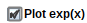Supply and Demand - Maple Help

Supply and Demand

 Main Concept Supply and demand are two fundamental concepts of economics. The law of demand states that as price increases, the quantity of items demanded by consumers decreases. The law of supply states that the higher the price, the greater quantity supplied by the manufacturers.   For given supply and demand curves, the market price and quantity of goods sold will fluctuate until they reach equilibrium at the point where the two curves intersect. At equilibrium, the number of goods demanded is the same as the number of goods that can be manufactured at that price.

The following graph shows simplified models of the supply (blue) and demand (green) curves. Use the sliders to adjust the parameters of the curves and observe how their point of intersection changes.

 Supply Minimum production cost per item ($):Maximum quantity (MaxQ) that can be produced at minimum average cost:Increase in average production cost per item for each extra item supplied above MaxQ ($):Demand Maximum Price Demanded ($):Price reduction required to sell one extra item ($):More MathApps